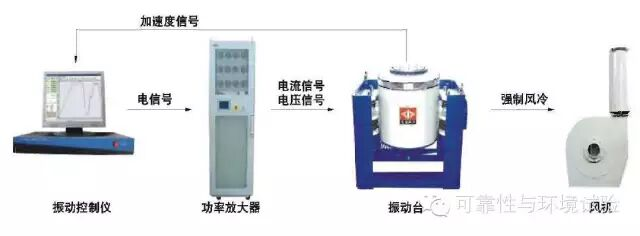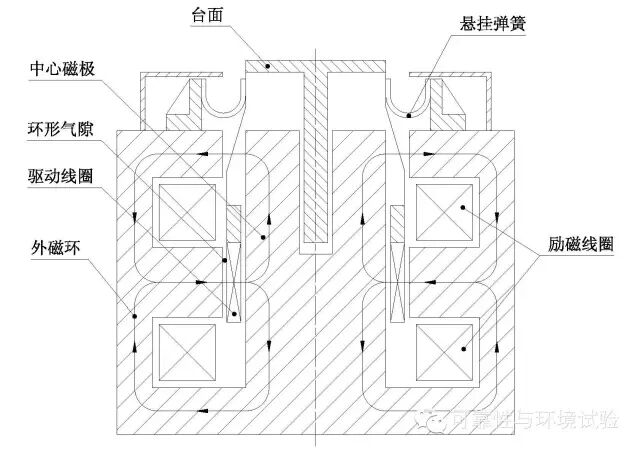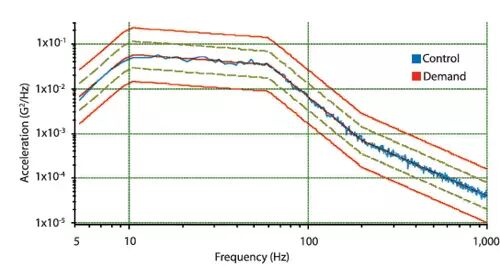# 如何判断振动台是否满足给出的要求？zui重要zui基本的一个公式：

f=ma

1、推力(f)  单位是牛（N）

2、质量(m) 单位是千克（kg）

1）试样的质量m1

2）夹具的质量m2

3）连接头的质量（一般是水平向试验时需要计入）m3

4）扩展台面的质量（水平滑台或者垂直扩展台面的质量）m4

5）动圈的质量（这是基本是一个恒定值）m5

3、试验要求的zui大加速度（a）单位：m/s^2或者g

Grms由ASD或者PSD通过复杂的计算得到。PSD曲线图例PSD曲线图例

1 g^2/Hz=(9.8 m/s^2)^2/Hz=96.04 m^2/s^3

4、位移，单位mm

5、频率范围，一般的电动振动台的频率虽然标称频率范围：2～2700Hz，但是实际一般工作频率在5~2500Hz范围，如果低于5Hz或者高于2500Hz就有可能做不了。

f=(m1+m2+m3+m4+m5+…)a/0.8

m1=1kg（试样）

m2=4kg（夹具）

m3=15kg（连接头，水平方向需要计入）

m40=50kg(垂直扩展台)

m41=300kg(水平台面)

m5=60kg（动圈）

X、Y向一般定义为水平向，Z向定义为垂直向。

f=(m1+m2+m3+m41+m5+…)a/0.8

=(1+4+15+300+60)*30/0.8

=14250kg

=142500N>80000N

f=(m1+m2+m40+m5+…)a/0.8

=(1+4+50+60)*30/0.8

=4312.5kg

=43125N<80000N

2021 版权所有 © 江西巨为仪器设备有限公司  备案号：赣ICP备19014404号-1 sitemap.xml 管理登陆 技术支持：化工仪器网400-1688-960